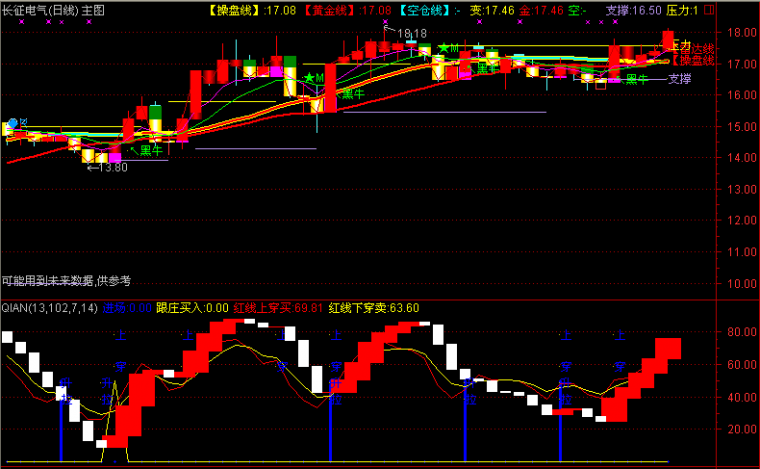﻿ 蓄势待发主图指标公式-通达信公式 －程序化交易（CXH99.COM）

# 蓄势待发主图指标公式[通达信公式]

EMA10:EMA(C,10);
EMA20:EMA(C,20);
EMA30:EMA(C,30);
EMA60:EMA(C,60);

M1:=1000*EMA10/EMA60<=1015 AND 1000*EMA10/EMA60>=975;
M2:=1000*EMA20/EMA60<=1020 AND 1000*EMA20/EMA60>=980;
M3:=1000*EMA30/EMA60<=1015 AND 1000*EMA30/EMA60>=985;
M4:=M1 AND M2 AND M3 AND CROSS(V0,EMA10);
M5:=M1 AND M2 AND M3 AND CROSS(V0,EMA30);
T1:=1000*EMA10/EMA30<=1010 AND 1000*EMA10/EMA30>=990;
T2:=1000*EMA20/EMA30<=1010 AND 1000*EMA20/EMA30>=990;
T3:=T1 AND T2 AND CROSS(V0,EMA10);
T4:=T1 AND T2 AND CROSS(V0,EMA30);
DRAWICON(M4 OR M5 OR T3 OR T4,L-0.10,9);
{程序化交易 www.cxh99.com }(注：由于人数限制，QQ或微信请选择方便的一个联系我们就行，加好友时请简单备注下您的需求，否则无法通过。谢谢您！)

【字体： 】【打印文章】【查看评论

没有相关内容